• PyTorch自动求导
2020-11-12 22:14:35

# 标量反向传播

当目标张量为标量时，backward()无需传入参数。

• 例子：假设 w , x , b w,x,b 都是标量， z = w x + b z=wx+b ，对标量 z z 调用backward()方法。

## 自动求导的主要步骤

import torch


### 1.定义叶子结点，算子节点

# 定义输入张量x
x = torch.Tensor()
# 设置前向传播
y = torch.mul(w,x)

False
True


### 2.查看叶子结点，非叶子结点的其他属性

# 查看非叶子结点y,z的requires_grad属性。
# 查看各节点是不是叶子节点
print(x.is_leaf)
print(y.is_leaf)
# 叶子结点：x,w,b
# 非叶子结点：y,z

True
True
False


### 3.自动求导，实现梯度反向传播

非叶子节点的梯度调用backward()之后，梯度将被清空。

# 基于z对张量进行反向传播，执行backward之后计算图会清空。
z.backward()
# 如果需要多次backward()需要设置参数retain_graph为True。此时梯度是累加的。
# z.backward(retain_graph=True)

# 查看叶子结点的梯度。

# 查看非叶子结点的梯度
# 非叶子节点的梯度调用backward()之后，梯度将被清空。故y,z此时没有梯度。


x的梯度是： None
w的梯度是： tensor([2.])


# 非标量反向传播

Pytorch只允许标量对张量进行求导

## 步骤

### 1.定义叶子结点，计算结点

import torch

# 定义叶子张量x，形状为1x2
print(x)
# 初始化雅可比矩阵
J = torch.zeros(2,2)
print(J)
# 初始化目标张量，形状为1x2
y = torch.zeros(1,2)
# 定义y与x之间的映射关系
# y1 = x1**2+3*x2
# y2 = x2**2+2*x1
y[0,0]=x[0,0]**2+3*x[0,1]
y[0,1]=x[0,1]**2+2*x[0,0]
print(y)

tensor([[2., 3.]], requires_grad=True)
tensor([0., 0.])


### 2.调用backward()获取y对x的梯度

需要重复使用backward()时,retain_graph=True

# 生成y1对x的梯度
y.backward(torch.Tensor([[1,0]]),retain_graph=True)
# 因为梯度是累加的，所以需要清除对x的梯度
# 生成y2对x的梯度
y.backward(torch.Tensor([[0,1]]))
# 雅可比矩阵的值
print(J)

tensor([[4., 3.],
[2., 6.]])深度学习 神经网络
更多相关内容
• 今天小编就为大家分享一篇关于PyTorch 自动求导机制详解，具有很好的参考价值，希望对大家有所帮助。一起跟随小编过来看看吧

1.求导

params = torch.tensor([1.0, 0.0], requires_grad=True)

注意到了张量构造函数的 require_grad = True 吗?这个参数告诉PyTorch需要追踪在 params 上进行运算而产生的所有张量。换句话说，任何以 params 为祖先的张量都可以访问从 params 到该张量所调用的函数链。如果这些函数是可微的（大多数PyTorch张量运算都是可微的），则导数的值将自动存储在参数张量的 grad 属性中。

警告：PyTorch的新手（以及很多经验丰富的人）经常忽视的事情：是积累（accumulate）而不是存储（store）。

为防止这种情况发生，你需要在每次迭代时将梯度显式清零。可以使用就地方法 zero_ 轻松地做到这一点：

if params.grad is not None:
params.grad.zero_()# 这可以在调用backward之前在循环中的任何时候完成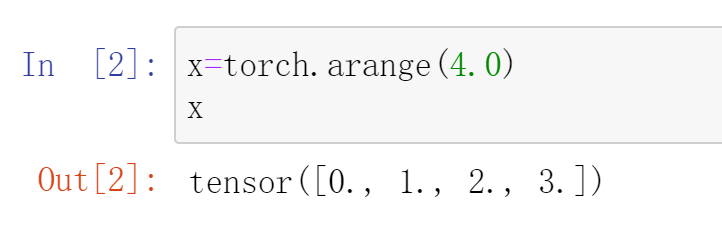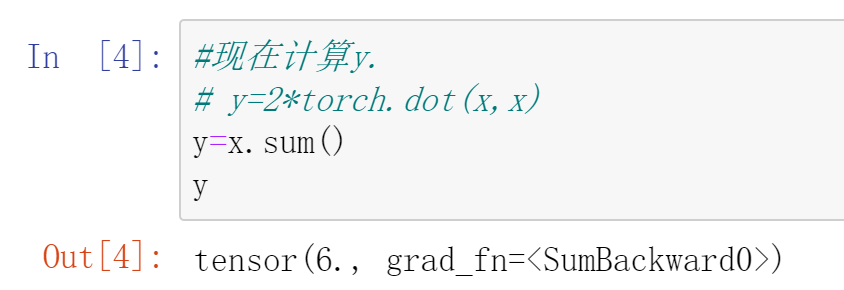第一次求导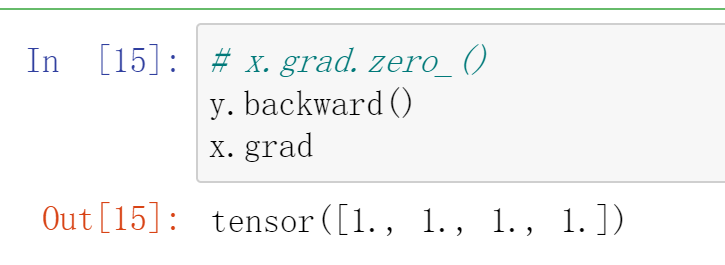第二次求导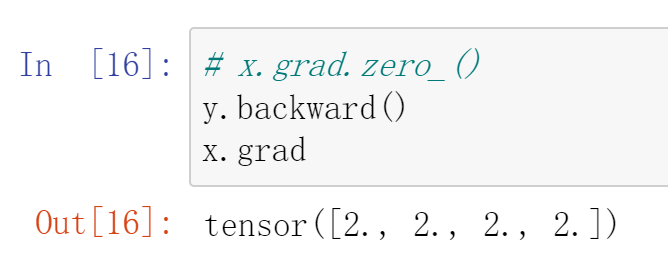求和的梯度为1，但是梯度不归0就累计了

请注意，更新参数时，你还执行了奇怪的 .detach().requires_grad_() 。要了解原因，请考虑一下你构建的计算图。为了避免重复使用变量名，我们重构 params 参数更新行： p1 = (p0 * lr *

2.优化

设置损失函数:均方差损失函数

def loss_fn(t_p, t_c):
squared_diffs = (t_p - t_c)**2
return squared_diffs.mean()

现在创建参数并实例化一个梯度下降优化器：

t_p = model(t_u, *params)
loss = loss_fn(t_p, t_c)
loss.backward()
optimizer.step()
params

调用 step 后 params 的值就会更新，无需亲自更新它！调用 step 发生的事情是：优化器通过将
params 减去 learning_rate 与 grad 的乘积来更新的 params ，这与之前手动编写的更新过程完全相同。

params = (params - learning_rate *params.grad).detach().requires_grad_()

params = torch.tensor([1.0, 0.0], requires_grad=True)
learning_rate = 1e-2
optimizer = optim.SGD([params], lr=learning_rate)
t_p = model(t_un, *params)
loss = loss_fn(t_p, t_c)
loss.backward()
optimizer.step()
params

for循环开始

def training_loop(n_epochs, learning_rate, params, t_u, t_c):
for epoch in range(1, n_epochs + 1):
t_p = model(t_u, *params)
loss = loss_fn(t_p, t_c)
loss.backward()
if epoch % 500 == 0:
print('Epoch %d, Loss %f' % (epoch, float(loss)))
return params

开始训练

t_un = 0.1 * t_u
training_loop(
n_epochs = 5000,
learning_rate = 1e-2,
t_u = t_un,
t_c = t_c)

优化器设置

params = torch.tensor([1.0, 0.0], requires_grad=True)
learning_rate = 1e-5
optimizer = optim.SGD([params], lr=learning_rate)

展开全文深度学习 机器学习
• ## pytorch自动求导机制

千次阅读 2022-01-24 00:01:21

在训练神经网络时，我们最常用的算法就是反向传播（BP）。

• 计算图是由节点和边组成的，其中的一些节点是数据，一些是数据之间的运算
• 计算图实际上就是变量之间的关系
• tensor 和 function 互相连接生成的一个有向无环图

# Tensors,Function,计算图

考虑最简单的例子，一个一层的神经网络

• input：x

• parameters：w，b

import torch

x = torch.ones(5)  # input tensor
y = torch.zeros(3)  # expected output
z = torch.matmul(x, w)+b #x 和 w 矩阵相乘，再加上 bias b
loss = torch.nn.functional.binary_cross_entropy_with_logits(z, y)


其计算图如下所示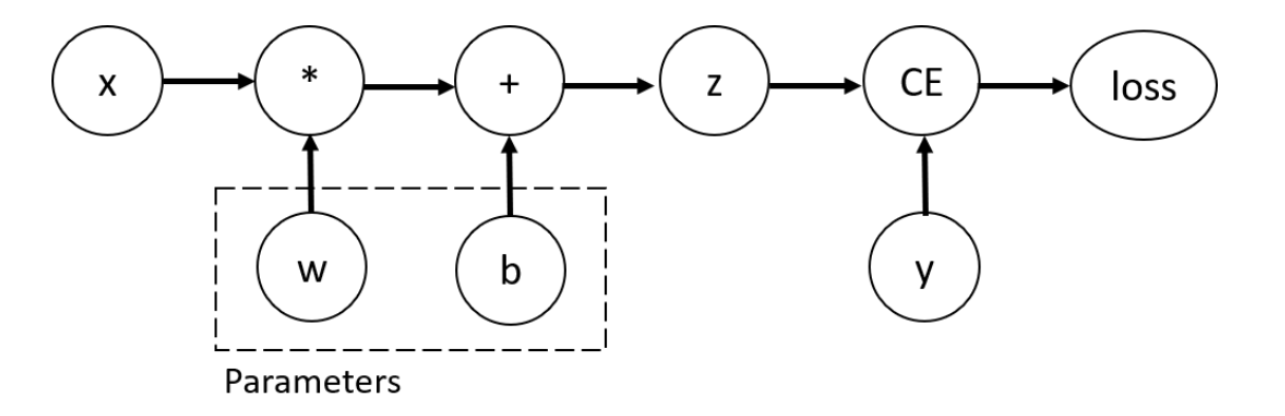• 我们可以在创建tensor时设置 requires_grad = True 来支持梯度计算

我们对 tensor 应用的来构建计算图的函数，实际上是 Function 类的一个对象。

• 该对象知道如何在前向传播中实施函数

• 也知道如何在反向传播中计算梯度

print('Gradient function for z =', z.grad_fn)
>>Gradient function for loss = <BinaryCrossEntropyWithLogitsBackward object at 0x000002A040C867F0>


# 计算梯度

为了更新参数，我们需要计算 loss function 关于参数的梯度。

• 我们使用 loss.backward() 来计算梯度

loss.backward()
>>tensor([[0.2832, 0.0843, 0.3005],
[0.2832, 0.0843, 0.3005],
[0.2832, 0.0843, 0.3005],
[0.2832, 0.0843, 0.3005],
[0.2832, 0.0843, 0.3005]])
>>tensor([0.2832, 0.0843, 0.3005])

• note：

• 由于性能原因，在给定的计算图中，我们仅能使用 backward() 计算梯度一次（每次backward之后，计算图会被释放，但叶子节点的梯度不会被释放。叶子节点就是参数，不包括一些运算产生的中间变量。比如 x，w是叶子节点，但是 h = wx，h就不是叶子节点）。如果需要多次计算，我们需要设置 backward 的 retain_graph = True，这样的话求得的最终梯度是几次梯度之和

x = torch.ones((1, 4), dtype=torch.float32, requires_grad=True)
y = x ** 2
z = y * 4
loss1 = z.mean()
loss2 = z.sum()
loss1.backward(retain_graph=True)
loss2.backward() #如果上面没有设置 retain_graph = True，这里会报错


# 禁用梯度跟踪

有时，我们只想对数据应用模型(forward过程)，而不考虑模型的更新，这时我们可以不使用梯度跟踪

z = torch.matmul(x, w)+b
>>True

z = torch.matmul(x, w)+b
>>False

• 另一种方式是使用 detach()

z = torch.matmul(x, w)+b
z_det = z.detach()
>>False


禁用梯度跟踪的一些原因

• fine-tune一个预训练的模型时，我们需要冻结模型的一些参数
• 当进行前向传播时，加速计算。对不追踪梯度的tensor进行计算会更高效

# 计算图的扩展

• 运行请求的操作来计算结果张量
• 在DAG中保持操作的梯度函数

反向传播过程开始，当 .backward() 在 DAG 根上被使用时

• 将它们累加到各个 tensor 的 .grad 属性中
• 利用链式法则，一直传播到叶子 tensor

DAGs 在 pytorch 中是动态的。值得注意的是，图是从头创建的。在每次.backward()调用之后autograd开始填充一个新的图。这正是允许我们在模型中使用控制流语句的原因，如果需要的话，我们可以在每次迭代中改变 shape（tensor的属性），size（tensor的方法） 和 operations（加减乘除等运算过程）

# Tensor梯度和雅克比乘法

在很多情况下，我们loss function的结果是一个标量，我们以此来计算参数的梯度。但是，有些时候，我们的 loss 是 tensor。在这种情况下，pytorch 允许我们计算所谓的雅克比乘法，而不是梯度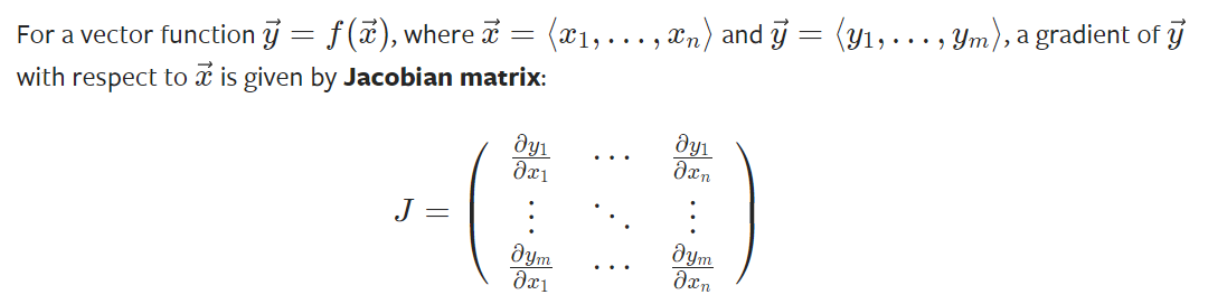• 如果是(向量)矩阵对(向量)矩阵求导(tensor对tensor求导)，实际上是先求出Jacobian矩阵中每一个元素的梯度值(每一个元素的梯度值的求解过程对应上面的计算图的求解方法)，然后将这个Jacobian矩阵与grad_tensors参数对应的矩阵相乘，得到最终的结果。v中的每个值代表该位置对应输出产生的梯度的权重。因此 v 的大小与输出 y 相同

• 当y 和 x 都是向量时，雅克比点乘 v T ⋅ J v^T \cdot J 是矩阵乘法， v = ( v 1 , … , v m ) v = (v_1,\dots,v_m) v v 是 backward函数的参数，与 y 的大小一致。 v v 中的每个量代表该位置对应 y 元素产生梯度的权重

x1 = torch.tensor(1, requires_grad=True, dtype = torch.float)
x2 = torch.tensor(2, requires_grad=True, dtype = torch.float)
x3 = torch.tensor(3, requires_grad=True, dtype = torch.float)
y = torch.randn(3)
y = x1 ** 2 + 2 * x2 + x3
y = x1 + x2 ** 3 + x3 ** 2
y = 2 * x1 + x2 ** 2 + x3 ** 3
v = torch.tensor([3, 2, 1], dtype=torch.float)
y.backward(v)

>>tensor(10.)
>>tensor(34.)
>>tensor(42.)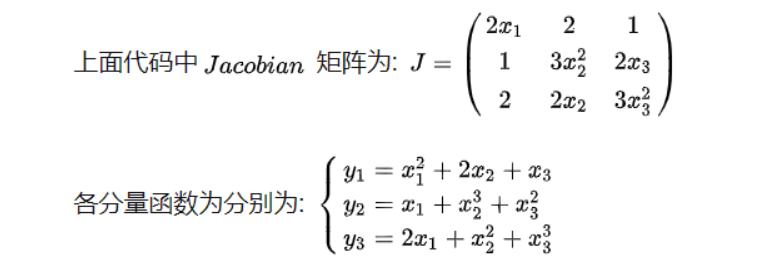v ∘ J = [ 3 ∗ 2 x 1 + 2 ∗ 1 + 1 ∗ 2 , 3 ∗ 2 + 2 ∗ 3 x 2 2 + 1 ∗ 2 x 2 , 3 ∗ 1 + 2 ∗ 2 x 3 + 1 ∗ 3 x 3 2 ] = [ 10 , 34 , 42 ] v \circ J=\left[3 * 2 x_{1}+2 * 1+1 * 2,3 * 2+2 * 3 x_{2}^{2}+1 * 2 x_{2}, 3 * 1+2 * 2 x_{3}+1 * 3 x_{3}^{2}\right]=[10,34,42]

可以理解为

$v \circ J = 3 * [2x_1\,2\,1] + 2 * [1\,3x_2^2\,2x_3] + 1 * [2\,2x_2\,3x_3^2]$

- 第一项是 $y_1$ 产生的梯度，系数为 3
- 第二项是 $y_2$ 产生的梯度，系数为 2
- 第三项是 $y_3$ 产生的梯度，系数为 1

• 矩阵对矩阵，对应位置相乘（点乘，也是加权求和）v的大小与输出相同，每个值代表对应位置产生梯度的权重，然后相加

• 输出是 3 * 3 的，一共 9 个输出
• 每个输出对参数都有一个梯度矩阵，总的梯度是九个矩阵的加权
inp = torch.tensor([[1., 0, 0], [0, 1, 0], [0, 0, 1], [1, 1, 1], [1, 1, 1]])
out = torch.matmul(w, inp)
print(out)
>>tensor([[3., 3., 3.],
[3., 3., 3.],

x = torch.tensor([[1, 0, 0], [0, 0, 0], [0, 0, 0]]) #只考虑第一个输出产生的梯度，该梯度是一个矩阵
out.backward(x, retain_graph=True)
>>  tensor([[1., 0., 0., 1., 1.],
[0., 0., 0., 0., 0.],
[0., 0., 0., 0., 0.]])

x = torch.ones(3, 3)
out.backward(x) # 所有输出产生的梯度权重都是 1，总的梯度为 9 个矩阵之和
>> tensor([[1., 1., 1., 3., 3.],
[1., 1., 1., 3., 3.],
[1., 1., 1., 3., 3.]])

• 输出是标量的时候，v 相当于 tensor(1.0) ，可以省略

展开全文深度学习 python
• Pytorch 自动求导机制 文章目录Pytorch 自动求导机制1. 简单的自动求导（输出是1维）2. 多维数组的自动求导（输出是多维）3. 多次自动求导 自动求导PyTorch 中非常重要的特性，能够让我们避免手动去计算非常复杂...

# Pytorch 自动求导机制

### 文章目录

自动求导是 PyTorch 中非常重要的特性，能够让我们避免手动去计算非常复杂的导数，这能够极大地减少了我们构建模型的时间。

每个Tensor都有个标志：requires_grad，它都允许从梯度计算中精细地排除子图，并可以提高效率。

requires_grad=True 要求梯度

requires_grad=False 不要求梯度

什么是排除子图？
答： 排除没必要的梯度计算。

requires_grad

如果有一个单一的输入操作需要梯度，它的输出也需要梯度。相反，只有所有输入都不需要梯度，输出才不需要。如果其中所有的变量都不需要梯度进行，后向计算不会在子图中执行。

步骤：

• 导入Variable并指定requires_grad=True,
• y.backward()
• x.grad

## 1. 简单的自动求导（输出是1维）

import torch

x = torch.Tensor()
# tensor 变成Variable

y=x**3

y.backward()

tensor([12.])


例2： z = ( x + 2 ) 2 + 3 z = (x + 2)^2 + 3 z z x x 进行求导。

x = Variable(torch.Tensor(), requires_grad=True)
y = x + 2
z = y ** 2 + 3
print(z)

tensor([19.], grad_fn=<AddBackward0>)

# 使用自动求导
z.backward()

tensor([8.])


例3：更复杂的例子

x = Variable(torch.randn(5, 10), requires_grad=True)

out = torch.mean(y - torch.mm(x, w)) # torch.matmul 是做矩阵乘法或者的使用torch.mm()
print(out)
out.backward()

tensor(-0.8683, grad_fn=<MeanBackward0>)

# 得到 x 的梯度

tensor([[ 0.0479,  0.0470,  0.0192,  0.1219,  0.2274, -0.1751,  0.0755,  0.0062,
-0.1455,  0.0992],
[ 0.0479,  0.0470,  0.0192,  0.1219,  0.2274, -0.1751,  0.0755,  0.0062,
-0.1455,  0.0992],
[ 0.0479,  0.0470,  0.0192,  0.1219,  0.2274, -0.1751,  0.0755,  0.0062,
-0.1455,  0.0992],
[ 0.0479,  0.0470,  0.0192,  0.1219,  0.2274, -0.1751,  0.0755,  0.0062,
-0.1455,  0.0992],
[ 0.0479,  0.0470,  0.0192,  0.1219,  0.2274, -0.1751,  0.0755,  0.0062,
-0.1455,  0.0992]])

# 得到 y 的的梯度

tensor([[0.0400, 0.0400, 0.0400, 0.0400, 0.0400],
[0.0400, 0.0400, 0.0400, 0.0400, 0.0400],
[0.0400, 0.0400, 0.0400, 0.0400, 0.0400],
[0.0400, 0.0400, 0.0400, 0.0400, 0.0400],
[0.0400, 0.0400, 0.0400, 0.0400, 0.0400]])

# 得到 w 的梯度

tensor([[0.0219, 0.0219, 0.0219, 0.0219, 0.0219],
[0.0160, 0.0160, 0.0160, 0.0160, 0.0160],
[0.0847, 0.0847, 0.0847, 0.0847, 0.0847],
[0.1473, 0.1473, 0.1473, 0.1473, 0.1473],
[0.0931, 0.0931, 0.0931, 0.0931, 0.0931],
[0.0924, 0.0924, 0.0924, 0.0924, 0.0924],
[0.1073, 0.1073, 0.1073, 0.1073, 0.1073],
[0.0393, 0.0393, 0.0393, 0.0393, 0.0393],
[0.0226, 0.0226, 0.0226, 0.0226, 0.0226],
[0.0419, 0.0419, 0.0419, 0.0419, 0.0419]])


## 2. 多维数组的自动求导（输出是多维）

例4：多维数组的自动求导机制

m = Variable(torch.FloatTensor([[2, 3]]), requires_grad=True) # 构建一个 1 x 2 的矩阵
n = Variable(torch.zeros(1, 2)) # 构建一个相同大小的 0 矩阵
print(m)
print(n)

tensor([[2., 3.]], requires_grad=True)
tensor([[0., 0.]])

# 通过 m 中的值计算新的 n 中的值
n[0, 0] = m[0, 0] ** 2
n[0, 1] = m[0, 1] ** 3
print(n)

tensor([[ 4., 27.]], grad_fn=<CopySlices>)

import numpy as np
a =np.array([[1,2,3],[4,5,6]])

a[1,1]==a #True
# 只有二维数组或矩阵，tensor才能这样取数

True


n = ( n 0 ,   n 1 ) = ( m 0 2 ,   m 1 3 ) = ( 2 2 ,   3 3 ) n = (n_0,\ n_1) = (m_0^2,\ m_1^3) = (2^2,\ 3^3) ,对 n n 进行反向传播，也就是 n n m m 的导数。
∂ n ∂ m = ∂ ( n 0 ,   n 1 ) ∂ ( m 0 ,   m 1 ) \frac{\partial n}{\partial m} = \frac{\partial (n_0,\ n_1)}{\partial (m_0,\ m_1)}

在 PyTorch 中，如果要调用自动求导，需要往backward()中传入一个参数，这个参数的形状和 n 一样大，比如是 ( w 0 ,   w 1 ) (w_0,\ w_1) ，那么自动求导的结果就是：
∂ n ∂ m 0 = w 0 ∂ n 0 ∂ m 0 + w 1 ∂ n 1 ∂ m 0 \frac{\partial n}{\partial m_0} = w_0 \frac{\partial n_0}{\partial m_0} + w_1 \frac{\partial n_1}{\partial m_0}
∂ n ∂ m 1 = w 0 ∂ n 0 ∂ m 1 + w 1 ∂ n 1 ∂ m 1 \frac{\partial n}{\partial m_1} = w_0 \frac{\partial n_0}{\partial m_1} + w_1 \frac{\partial n_1}{\partial m_1}

n.backward(torch.ones_like(n)) # 将 (w0, w1) 取成 (1, 1)

tensor([[ 4., 27.]])


关键：为什么要torch.ones_like(n)【向量求导】
n不是一个标量，是一个向量，需要传入一个维数一样的1向量，使得梯度是每个分量的求梯度的和。

验证：
∂ n ∂ m 0 = w 0 ∂ n 0 ∂ m 0 + w 1 ∂ n 1 ∂ m 0 = 2 m 0 + 0 = 2 × 2 = 4 \frac{\partial n}{\partial m_0} = w_0 \frac{\partial n_0}{\partial m_0} + w_1 \frac{\partial n_1}{\partial m_0} = 2 m_0 + 0 = 2 \times 2 = 4
∂ n ∂ m 1 = w 0 ∂ n 0 ∂ m 1 + w 1 ∂ n 1 ∂ m 1 = 0 + 3 m 1 2 = 3 × 3 2 = 27 \frac{\partial n}{\partial m_1} = w_0 \frac{\partial n_0}{\partial m_1} + w_1 \frac{\partial n_1}{\partial m_1} = 0 + 3 m_1^2 = 3 \times 3^2 = 27

## 3. 多次自动求导

通过调用backward 我们可以进行一次自动求导，如果我们再调用一次 backward，会发现程序报错，没有办法再做一次。这是因为 PyTorch 默认做完一次自动求导之后，计算图就被丢弃了，所以两次自动求导需要手动设置一个东西，我们通过下面的小例子来说明。

x = Variable(torch.FloatTensor(), requires_grad=True)
y = x * 2 + x ** 2 + 3
print(y)

tensor([18.], grad_fn=<AddBackward0>)


设置retain_graphTrue来保留计算图

y.backward(retain_graph=True)

tensor([8.])

y.backward() # 再做一次自动求导，这次不保留计算图

tensor([16.])


注意：这个张量的所有梯度将会自动累加到.grad属性.

y.backward() # 第三次做自动求导，这次不保留计算图


练习
x = [ x 0   x 1 ] = [ 2   3 ] x = \left[ \begin{matrix} x_0 \ x_1 \end{matrix} \right] = \left[ \begin{matrix} 2 \ 3 \end{matrix} \right]

k = ( k 0 ,   k 1 ) = ( x 0 2 + 3 x 1 ,   2 x 0 + x 1 2 ) k = (k_0,\ k_1) = (x_0^2 + 3 x_1,\ 2 x_0 + x_1^2)

求：
j = [ ∂ k 0 ∂ x 0 ∂ k 0 ∂ x 1   ∂ k 1 ∂ x 0 ∂ k 1 ∂ x 1 ] j = \left[ \begin{matrix} \frac{\partial k_0}{\partial x_0} & \frac{\partial k_0}{\partial x_1} \ \frac{\partial k_1}{\partial x_0} & \frac{\partial k_1}{\partial x_1} \end{matrix} \right]

x = Variable(torch.Tensor([2,3]),requires_grad=True)

k=Variable(torch.zeros(2))
k=x**2+3*x
k=2*x+x**2
print(k)

tensor([13., 13.], grad_fn=<CopySlices>)

j = torch.zeros(2, 2)

k.backward(torch.FloatTensor([1, 0]), retain_graph=True)

k.backward(torch.FloatTensor([0, 1]))
print(j)

tensor([[4., 3.],
[2., 6.]])

k.backward(torch.ones_like(k),retain_graph=True)


参考：自动求导机制

展开全文python
• 用numpy、PyTorch自动求导、torch.nn库实现两层神经网络，实现从手动求导到自动求导再到模型的一步步深入。神经网络 深度学习 人工智能 机器学习python
• 基于pytorch自动求导机制的牛顿迭代法实现框架，求解的函数可自行替换为任意函数
• 前言：构建深度学习模型的基本流程就是：...由损失函数求导的过程，称为“反向传播”，求导是件辛苦事儿，所以自动求导基本上是各种深度学习框架的基本功能和最重要的功能之一，PyTorch也不例外，后面有时间会写一下...
• 这里写自定义目录标题pytorch自动求导机制——代码实现代码实现 pytorch自动求导机制——代码实现 代码实现 import torch import torch.nn as nn import numpy as np #########————————定义模型————...深度学习
• 人工智能AI：Keras PyTorch MXNet TensorFlow PaddlePaddle 深度学习实战（不定时更新） 梯度下降和反向传播 目标 知道什么是梯度下降 知道什么是反向传播 1. 梯度是什么? 梯度：是一个向量，导数+变化最快的...人工智能
• 动态建图为什么可以反向自动求导？我对于这个问题一直存在疑惑，但是去网上有没有找到好的解释，于是，没办法，自己实验吧。首先，我们做如下定义：a = torch.randn(2)b = torch.randn(2)c = torch.randn(2,requires...python
• 使用PyTorch自动求导机制编写多元牛顿迭代法（PyTorch向量对向量求导）深度学习
• 沿着从根结点到叶结点的路径，利用链式法则就可以自动计算所有的梯度。 就内部而言， autograd 将此图表示为关于 Function 对象(实际上是表达式)的图， Function 可用于计算评估图的结果。当执行前向传播时， ...
• https://blog.csdn.net/qjk19940101/article/details/79557204 ... https://github.com/Roc-J/Pytorch_projects/blob/master/Neural_Networks/linear-regr...深度学习
• 由损失函数求导的过程，称为“反向传播”，求导是件辛苦事儿，所以自动求导基本上是各种深度学习框架的基本功能和最重要的功能之一，PyTorch也不例外，后面有时间会写一下tensorflow和mxnet框架的自动求导。...Autograd...